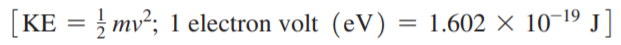×
Get Full Access to Chemistry: A Molecular Approach - 3 Edition - Chapter 7 - Problem 78e
Get Full Access to Chemistry: A Molecular Approach - 3 Edition - Chapter 7 - Problem 78e

×

# An X-ray photon of wavelength 0.989 nm strikes a surface.ISBN: 9780321809247 1

## Solution for problem 78E Chapter 7

Chemistry: A Molecular Approach | 3rd Edition

• Textbook Solutions
• 2901 Step-by-step solutions solved by professors and subject experts
• Get 24/7 help from StudySoup virtual teaching assistantsChemistry: A Molecular Approach | 3rd Edition

4 5 1 318 Reviews
12
2
Problem 78E

An X-ray photon of wavelength 0.989 nm strikes a surface. The emitted electron has a kinetic energy of 969 eV. What is the binding energy of the electron in kJ/mol?Step-by-Step Solution:
Step 1 of 3

Solution The balanced equation for the reactions are as given below (a)Chorine reacts with water Cl2(g)O 2C(l)+HOCl (aq) (aq) (b)Barium metal is heated in an atmosphere of hydrogen gas Ba +H BaH (s) 2(g) 2(s) (c)Lithium reacts with sulfur Li(s) (s) S 2 (s) (d)Fluorine reacts with magnesium metal. F +Mg MgF 2(g) (s) 2(s)

Step 2 of 3

Step 3 of 3

##### ISBN: 9780321809247

This full solution covers the following key subjects: reacts, Write, fluorine, nonmetals, equation. This expansive textbook survival guide covers 82 chapters, and 9454 solutions. Since the solution to 78E from 7 chapter was answered, more than 418 students have viewed the full step-by-step answer. This textbook survival guide was created for the textbook: Chemistry: A Molecular Approach, edition: 3. The full step-by-step solution to problem: 78E from chapter: 7 was answered by , our top Chemistry solution expert on 02/22/17, 04:35PM. The answer to “?An X-ray photon of wavelength 0.989 nm strikes a surface. The emitted electron has a kinetic energy of 969 eV. What is the binding energy of the electron in kJ/mol?” is broken down into a number of easy to follow steps, and 30 words. Chemistry: A Molecular Approach was written by and is associated to the ISBN: 9780321809247.

Unlock Textbook Solution